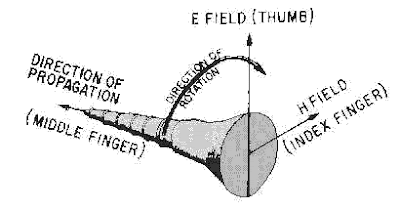## söndag 13 november 2011

### The Poynting VectorThe Poynting vector $E\times H$ representing the energy flux of an electromagnetic field.

Mathematical Physics of Blackbody Radiation gives a new wave based proof of Planck's radiation law in the form
• $Radiation + Heating = Forcing$,
where
• $Forcing = \int\int f^2(x,t)\, dxdt$
with $f(x,t)$ the amplitude of the incoming wave.

The measure of $Forcing$ as the (integral of the) square $f^2$ of the amplitude $f$ connects to the Poynting vector $E\times H$ representing the energy flux of an electromagnetic field with $E$ the electric field and $H$ the magnetic field having the same amplitude.